# Think-a-Dot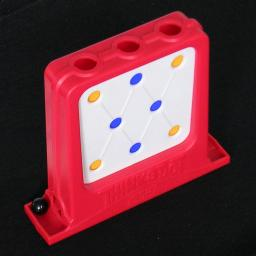Think-a-Dot has an upright frame with three openings at the top, into which you can drop a marble. The marble travels through and drops out the bottom. There are eight dots on the front of the frame, in rows of three, two, and three, and they are yellow or blue. They indicate the state of a flip-flop gate inside the frame. If a marble reaches a gate, it is deflected either left or right depending on the state of the gate, and as the marble passes, the gate will flip over to the other state.

At the start you tilt the frame to the right (or left), so that all the gates face the same way. The four corner gates are coloured differently than the rest, so the corner dots will be yellow, and the rest blue (or vice versa if you tilted it to the left). Given a particular target pattern of dots, the aim is to drop the marble into the holes at the top until the target pattern is reached, preferably in as few moves as possible.

This puzzle was made by E.S.R. Inc. in around 1966. Its patent, US 3,388,483, was filed by Joseph A. Weisbecker in July 21, 1966, and granted June 18, 1968. The letters E.S.R. stand for Education Science Research (not Edmund Scientific as is sometimes thought) and they made several fascinating contraptions:

 Name Patent, filing date Description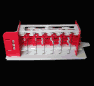Digi-Comp 1 US 3,273,794, Oct 21 1963 A mechanical model of a 3-bit computer, using sliding plates, programmable by attaching rods to make parts interact.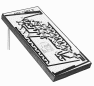Digi-Comp 2 US 3,390,471, Apr 30 1965 A mechanical model that does binary arithmetic (add, subtract, multiply, divide, complement and negate), using marbles and flip-flop gates. It looks like a pinball machine.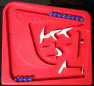Dr. Nim Also US 3,390,471 A mechanical Nim playing machine, using 15 marbles and three flip-flop gates.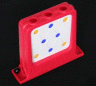Think-a-Dot US 3,388,483, Jul 21 1966 A mechanical puzzle, using one marble and 8 flip-flop gates.

If your browser supports it, you can click on the link below to play with a Javascript version of Think-a-Dot.

## The number of positions:

The eight gates can each be in two positions, giving at most 28 patterns. There is a parity restriction - every move will change one top row gate and one bottom row gate, so the number of blue or yellow dots in the top and bottom rows together will always remain even. This means that there are really only 27=128 positions.

## Analysis:

Much of what follows below is based on the texts on the Fractions-plus site (now to be found at docstoc).

## The order of the moves

I will label the three openings at the top A, B, and C.

Every move changes the state of some gates, which in turn changes what the next move will do. You would therefore initially expect the order in which you do moves to matter. The amazing thing is however that it doesn't. So A followed by B is the same as B and then A. Similarly B C is the same as C B. The reason for this is clear if you imagine the two moves being done simultaneously. If the marbles are at different gates in some row, then their order is obviously unimportant because those two gates are independent of each other. If at some row the two marbles have to go through the same gate, then again it doesn't matter which marble goes through first, as the marbles are identical. One marble will be deflected one way, the second the other way, but it doesn't matter which is which. Their combined effects are the same regardless of the ordering.

The move order is immaterial, so lets simply represent them by three numbers, the number of marbles that are dropped into each column. A triple (a,b,c) means that a marbles are dropped in column A, b in column B, and c in column C.

## Repeating moves

If we drop two marbles in a column, the first row will be unaffected. This is because the second marble undoes the first row flip that the first marble did. Those two marbles will, however, take different paths when passing through the remaining rows and so have different effects there. If we drop another two marbles in the same column, the first row is unaffected still, but now they undo the effect the first two marbles had on the second row. If we now double the number of marbles again, putting a total of 8 marbles in the same column, then even the third row will be unaffected. This shows straight away that you need never put eight or more marbles in any one column, and the worst case certainly won't need more than 7+7+7=21 moves. As we shall see later, the worst case is actually 9 moves.

The previous paragraph suggests that we can solve the puzzle row by row, first solving the top row with single moves, then the second row with double moves, and finally the last row with quadruple moves. We simply have to find out how the double moves (2,0,0), (0,2,0), and (0,0,2) affect the middle row, and how quadruple moves affect the last row. This leads to this strategy:

## Solution method 1:

1. Solve the top row by dropping a marble into the column of each incorrect dot.
2. Solve the middle row as follows:
1. To change only the left dot, do (2,0,0).
2. To change both dots, do (0,2,0).
3. To change only the right dot, do (0,0,2).
3. Solve the bottom row as follows:
1. To change the left and middle dots, do (4,0,0).
2. To change the left and right dots, do (0,4,0).
3. To change the middle and right dots, do (0,0,4).
4. If you need to change an odd number of dots on the bottom row, this is impossible. The pattern you are trying to create is unreachable from the current position.

This method is quite efficient, taking at most 9 moves. There are in fact some positions that cannot possibly be solved in fewer, so in those cases this method is optimal. It is however not optimal for all positions, as there are some that can be solved in fewer moves than this method uses.

## Binary counters

There is another way to see that repeating a move 8 times will have no effect. Take a look at any three gates that a single marble might go through if you put it in column B. For example, take the diagonal from column B to the left of the bottom row. The state of some of those three gates changes by dropping the marble into column B. If you try it out you will see that the three gates change the same way as a three-bit binary counter does, counting from 000 to 111, and then wrapping back to 000. A gate represents a 1 if it would let the marble through to the next gate in the path, and a 0 otherwise. The three gates will therefore be back to their initial state after 8 moves. This actually holds for any such path of three gates (even for the path along all the leftmost gates, or the one along all the rightmost gates, which behave slightly differently), so after 8 identical moves everything will be back to the initial state. This suggests the following very simple solution:

## Solution method 2:

1. If the top left dot is incorrect, drop a marble in column A.
2. Note the three dots on the diagonal from the top of column B to the bottom left. Drop marbles in column B until those three dots are correct.
3. Note the three dots on the diagonal from the top of column C to the bottom middle. Drop marbles in column C until those three dots are correct.
4. The last dot in the bottom row is determined by the parity restriction, so it should be correct, or else the pattern you are trying to make is not possible from the position you started with.

In the above method the worst case is (1,7,7) which has 15 moves. This is clearly not as efficient as the first method, but it is easier to remember.

## Optimal solving

Consider the moves (2,2,2). If you imagine 6 marbles dropping at the same time, two in each column, then you will see that the first row of dots will obviously not change, but neither will the second row because each gate gets two marbles, one arriving from the left and one from the right. The same goes for the last row, it also does not change. So regardless of the current state of the dots/gates, the moves (2,2,2) will have no effect.

We can use (2,2,2) to create better solutions. Suppose we found that the moves (3,2,6) created the pattern we want. If we apply (2,2,2) to it we still have the same pattern, but we have done (5,4,8) all together. We know that 8 times the same move has no effect either, so this is the same as (5,4,0). So the pattern we got by doing the 11 moves (3,2,6) can also be created by the 9 moves (5,4,0). We can apply (2,2,2) again to get (7,6,2), and again to get (9,8,4) = (1,0,4), and once more to get back to (3,2,6) which is where we started.
We now have four alternative solutions (3,2,6), (5,4,0), (7,6,2), (1,0,4), of which the last one is the best with only 5 moves.

Given any triple (a,b,c) with a,b,c<8, we get four triples that have the same effect - the triple itself, and three more by adding (2,2,2) one, two or three times. There are 83 triples, so these form 83/4 = 128 sets of equivalent triples. As there are also 128 reachable positions, such a set forms a complete set of solutions for a position. If we therefore have one solution for a pattern we can generate the set of four solutions, and if we then choose the best of those it will certainly be the optimal solution to that pattern.

An optimal triple will have the following properties:

1. Its largest number is smaller than 6.
If some number were 6 or larger, we could add (2,2,2) to get a better triple.
2. Its smallest number is smaller than 2.
If all numbers were 2 or larger, we could subtract (2,2,2) (the equivalent of adding it three times) to get a better triple.
3. Its medium number is smaller than 4.
If its medium number were 4 or larger, then there are at least two numbers that are 4 or more. We could then add (4,4,4) to get a better triple.

The largest number of an optimal triple is at most 5, the medium largest at most 3, and the smallest at most 1. So an optimal triple will never have more than 1+3+5=9 moves. Furthermore, triples such as (1,3,5) are actually optimal, so the worst case positions of Think-a-Dot have 9 moves.

## Mental optimal solving

It would be nice to be able to look at a dot pattern and be able to figure out an optimal solution without doing any moves before hand. Neither of the previous solution methods are suitable, since they have decisions based on the state of the dots after some moves have already been done. To eliminate this, we can use a variation of the second method.

## Solution method 3:

1. If the top middle dot is incorrect, drop a marble in column B.
2. Note the three leftmost dots of the puzzle. Drop marbles in column A until those three dots are correct.
3. Note the three rightmost dots of the puzzle. Drop marbles in column C until those three dots are correct.
4. The middle dot in the bottom row is determined by the parity restriction, so it should be correct, or else the pattern you are trying to make is not possible from the position you started with.

Where this method scores above method 2 is that steps b and c are independent. The state of the leftmost dots is not affected by C moves, and the state of the rightmost dots is not affected by A moves. You only have to be able to look ahead at most one move - once you know the dot pattern after step a, you can deduce the number of marbles needed in columns A and C. In fact, even this amount of look ahead is not necessary. Using binary arithmetic, we can calculate everything.

## Solution method 4: Mental optimal solution

1. Imagine the target pattern. Read the leftmost dots of the target pattern from bottom to top as a binary number, where a gate that deflects to the left is 0 and gate that deflects to the right is 1. The initial starting position with the colours Yellow, Blue, Yellow is therefore 0. In other words:
2. If the bottom left dot of the target pattern is blue, add 4, else leave it as 0.
3. If the middle left dot is yellow, then add 2 to your number.
4. If the top left dot is blue, then add 1 to your number.
Remember this result, which will be referred to as Lefttarget.
2. Do the same for the current pattern, creating a number Leftcurrent.
3. Calculate (Lefttarget-Leftcurrent)×5 (modulo 8). This calculation is modulo 8, which means that you have to add or subtract multiples of 8 to get a number in the range 0 to 7 inclusive. This is the number of marbles that need to be dropped in column A for the three leftmost dots to match.
4. Now do step b for the rightmost dots of the target pattern, resulting in a number Righttarget.
5. Also do the same for the rightmost dots of the current pattern, resulting in a number Rightcurrent.
6. Calculate (Righttarget-Rightcurrent)×3 (modulo 8). This is the number of marbles that need to be dropped in column C for the three rightmost dots to match.
7. We now have a triple (a,b,c) = ((Lefttarget-Leftcurrent)×5, 0, (Righttarget-Rightcurrent)×3 ), reduced modulo 8 so that all three numbers lie between 0 and 7 inclusive. This triple solves everything except maybe for the top middle (and bottom middle) dots.
If the top middle dot has to be changed from blue to yellow, then set b to 1 and add 2 to a (reducing it modulo 8 if necessary).
If the top middle dot has to be changed from yellow to blue, then set b to 1 and add 2 to c (reducing it modulo 8 if necessary).
8. The solution triple might not be optimal at this point, so do the following steps to make it so:
1. If two or more of the triple's numbers are 4 or larger, then subtract (or add) 4 from all three numbers to get a better solution triple.
2. If all three numbers are 2 or larger, then subtract 2 from all of them to get a better solution triple.
3. If one or more of the triple's numbers are 6 or 7, then add 2 (or subtract 6) from all three numbers to get a better solution triple.
You now have an optimal solution triple for getting to the target pattern.

Example: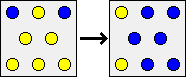Suppose the current position has blue dots at the top left and top right, and the other 6 dots are yellow. Suppose that our target pattern has yellow dots at the top left and bottom left and blue dots everywhere else.

1. The left dots of the target are Yellow/Blue/Yellow. This is exactly the same as the 'tilt right' position, so its left column reads as binary 000, simply 0.
2. The left dots of the current position are YYB from bottom to top. This differs from YBY in the last two spots so it represents 011 in binary, or 2+1=3 in decimal.
3. Now we calculate the difference times 5, to get (0-3)×5 = -15, which is 1 modulo 8. Therefore the first number of our triple is 1.
4. The right dots of the target are BBB, which when compared to YBY gives 101 in binary, or 4+1=5 in decimal.
5. The right dots of the current position are YYB, which when compared to YBY gives 011 in binary, or 2+1=3 in decimal.
6. Calculating the difference times 3, we get (5-3)×3 = 6. Therefore the third number of our triple is 6.
7. The top middle dot has to change from yellow to blue, so we would need to drop a marble in the middle column. This deflects the marble to the right, so we have to adjust the number for the right hand side by adding 2. Our triple becomes (1,1,6+2) = (1,1,8) = (1,1,0).
8. The triple (1,1,0) is obviously already optimal, so we can reach the target by dropping the marble once on the left and once in the middle.